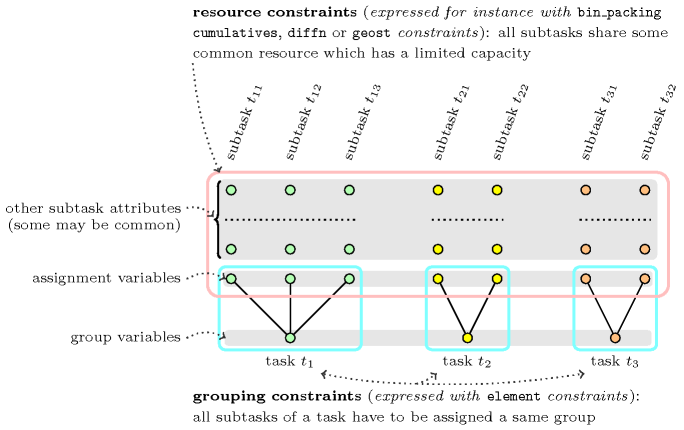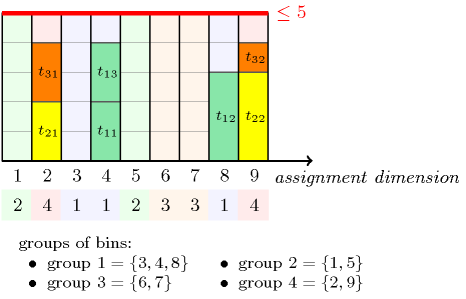### 3.7.18. Assignment to the same set of values

modelling: assignment to the same set of values Given several mutually disjoint finite sets of values ${𝒮}_{1},{𝒮}_{2},\cdots ,{𝒮}_{m}$ $\left(m>1\right)$ such that ${S}_{1}\cup {S}_{2}\cup \cdots \cup {S}_{m}=\left\{1,2,\cdots ,p\right\}$, as well as a set of variables ${V}_{1},{V}_{2},\cdots ,{V}_{n}$, the assignment to the same set of values subproblem consists of assigning all variables ${V}_{1},{V}_{2},\cdots ,{V}_{n}$ values that belong to the same set ${𝒮}_{i}$ $\left(1\le i\le m\right)$. As we will see later on, this subproblem arises naturally in many resource assignment problems where an additional constraint between variables ${V}_{1},{V}_{2},\cdots ,{V}_{n}$ also has to hold. The subproblem can be modelled as a conjunction of $\mathrm{𝚎𝚕𝚎𝚖𝚎𝚗𝚝}$ constraints of the form:

$\mathrm{𝚎𝚕𝚎𝚖𝚎𝚗𝚝}$$\left({V}_{1},〈\mathrm{𝑠𝑒𝑡}_\mathrm{𝑜𝑓}_{\mathrm{𝑣𝑎𝑙}}_{1},\mathrm{𝑠𝑒𝑡}_\mathrm{𝑜𝑓}_{\mathrm{𝑣𝑎𝑙}}_{2},\cdots ,\mathrm{𝑠𝑒𝑡}_\mathrm{𝑜𝑓}_{\mathrm{𝑣𝑎𝑙}}_{p}〉,\mathrm{𝑆𝐸𝑇}_\mathrm{𝐼𝑁𝐷𝐸𝑋}\right)\wedge$

$\mathrm{𝚎𝚕𝚎𝚖𝚎𝚗𝚝}$$\left({V}_{2},〈\mathrm{𝑠𝑒𝑡}_\mathrm{𝑜𝑓}_{\mathrm{𝑣𝑎𝑙}}_{1},\mathrm{𝑠𝑒𝑡}_\mathrm{𝑜𝑓}_{\mathrm{𝑣𝑎𝑙}}_{2},\cdots ,\mathrm{𝑠𝑒𝑡}_\mathrm{𝑜𝑓}_{\mathrm{𝑣𝑎𝑙}}_{p}〉,\mathrm{𝑆𝐸𝑇}_\mathrm{𝐼𝑁𝐷𝐸𝑋}\right)\wedge$

$\cdots$

$\mathrm{𝚎𝚕𝚎𝚖𝚎𝚗𝚝}$$\left({V}_{n},〈\mathrm{𝑠𝑒𝑡}_\mathrm{𝑜𝑓}_{\mathrm{𝑣𝑎𝑙}}_{1},\mathrm{𝑠𝑒𝑡}_\mathrm{𝑜𝑓}_{\mathrm{𝑣𝑎𝑙}}_{2},\cdots ,\mathrm{𝑠𝑒𝑡}_\mathrm{𝑜𝑓}_{\mathrm{𝑣𝑎𝑙}}_{p}〉,\mathrm{𝑆𝐸𝑇}_\mathrm{𝐼𝑁𝐷𝐸𝑋}\right)$,

where $\mathrm{𝑠𝑒𝑡}_\mathrm{𝑜𝑓}_{\mathrm{𝑣𝑎𝑙}}_{i}=j$ if and only if $i\in {𝒮}_{j}$ (i.e., $\mathrm{𝑠𝑒𝑡}_\mathrm{𝑜𝑓}_{\mathrm{𝑣𝑎𝑙}}_{i}$ corresponds to the index of the set that contains value $i$). The $k$-th $\mathrm{𝚎𝚕𝚎𝚖𝚎𝚗𝚝}$ constraint expresses that variable ${V}_{k}$ is assigned a value in set ${𝒮}_{\mathrm{𝑆𝐸𝑇}}_\mathrm{𝐼𝑁𝐷𝐸𝑋}$. Since all $\mathrm{𝚎𝚕𝚎𝚖𝚎𝚗𝚝}$ constraints share the same third argument this forces all variables ${V}_{1},{V}_{2},\cdots ,{V}_{n}$ to be assigned a value within the same set. Note that this conjunction of $\mathrm{𝚎𝚕𝚎𝚖𝚎𝚗𝚝}$ constraints corresponds to a Berge-acyclic constraint network. Consequently, one can achieve arc-consistency on this subproblem provided that arc-consistency is enforced on each $\mathrm{𝚎𝚕𝚎𝚖𝚎𝚗𝚝}$ constraint.

As an example, consider the four sets of values ${𝒮}_{1}=\left\{3,4,8\right\}$, ${𝒮}_{2}=\left\{1,5\right\}$, ${𝒮}_{3}=\left\{6,7\right\}$, and ${𝒮}_{4}=\left\{2,9\right\}$, as well as four variables $w$, $x$, $y$ and $z$ that all must be assigned values that belong to the same set ${𝒮}_{s}$ $\left(1\le s\le 4\right)$. This leads to the following conjunction of $\mathrm{𝚎𝚕𝚎𝚖𝚎𝚗𝚝}$ constraints:

$\mathrm{𝚎𝚕𝚎𝚖𝚎𝚗𝚝}$$\left(w,〈2,4,1,1,2,3,3,1,4〉,s\right)\wedge$

$\mathrm{𝚎𝚕𝚎𝚖𝚎𝚗𝚝}$$\left(x,〈2,4,1,1,2,3,3,1,4〉,s\right)\wedge$

$\mathrm{𝚎𝚕𝚎𝚖𝚎𝚗𝚝}$$\left(y,〈2,4,1,1,2,3,3,1,4〉,s\right)\wedge$

$\mathrm{𝚎𝚕𝚎𝚖𝚎𝚗𝚝}$$\left(z,〈2,4,1,1,2,3,3,1,4〉,s\right)$.

The first entry of the table $〈2,4,1,1,2,3,3,1,4〉$ is set to 2 since value 1 belongs to ${𝒮}_{2}$. Similarly, the second entry of the table is set of 4 since value 2 belongs to ${𝒮}_{4}$. The same logic is used for building up the other entries of the table.

A generalisation of this subproblem consists in lifting the restriction that the sets of values ${𝒮}_{1},{𝒮}_{2},\cdots ,{𝒮}_{m}$ are mutually disjoint. The only change to adapt the previous model is to replace within each $\mathrm{𝚎𝚕𝚎𝚖𝚎𝚗𝚝}$ constraint each value ${\mathrm{𝑣𝑎𝑙}}_{i}$ $\left(1\le i\le p\right)$ by a value variable ${\mathrm{𝑉𝑎𝑙}}_{i}$ (i.e., each value of a value variable represents a set containing $i$), where $j\in \mathrm{𝑑𝑜𝑚}\left({\mathrm{𝑉𝑎𝑙}}_{i}\right)$ if and only if $i\in {𝒮}_{j}$. Distinct $\mathrm{𝚎𝚕𝚎𝚖𝚎𝚗𝚝}$ constraints will get distinct value variables. As an example, consider the previous four sets of values where we add value 2 to ${𝒮}_{1}$ and value 5 to ${𝒮}_{3}$. We now have the sets ${𝒮}_{1}=\left\{2,3,4,8\right\}$, ${𝒮}_{2}=\left\{1,5\right\}$, ${𝒮}_{3}=\left\{5,6,7\right\}$, and ${𝒮}_{4}=\left\{2,9\right\}$ where value 2 occurs both in ${𝒮}_{1}$ and ${𝒮}_{4}$, and value 5 appears both in ${𝒮}_{2}$ and ${𝒮}_{3}$. This leads to the following conjunction of constraints:

$\mathrm{𝚒𝚗}$$\left({a}_{1},〈1,4〉\right)\wedge$$\mathrm{𝚒𝚗}$$\left({b}_{1},〈2,3〉\right)\wedge$$\mathrm{𝚎𝚕𝚎𝚖𝚎𝚗𝚝}$$\left(w,〈2,{a}_{1},1,1,{b}_{1},3,3,1,4〉,s\right)\wedge$

$\mathrm{𝚒𝚗}$$\left({a}_{2},〈1,4〉\right)\wedge$$\mathrm{𝚒𝚗}$$\left({b}_{2},〈2,3〉\right)\wedge$$\mathrm{𝚎𝚕𝚎𝚖𝚎𝚗𝚝}$$\left(x,〈2,{a}_{2},1,1,{b}_{2},3,3,1,4〉,s\right)\wedge$

$\mathrm{𝚒𝚗}$$\left({a}_{3},〈1,4〉\right)\wedge$$\mathrm{𝚒𝚗}$$\left({b}_{3},〈2,3〉\right)\wedge$$\mathrm{𝚎𝚕𝚎𝚖𝚎𝚗𝚝}$$\left(y,〈2,{a}_{3},1,1,{b}_{3},3,3,1,4〉,s\right)\wedge$

$\mathrm{𝚒𝚗}$$\left({a}_{4},〈1,4〉\right)\wedge$$\mathrm{𝚒𝚗}$$\left({b}_{4},〈2,3〉\right)\wedge$$\mathrm{𝚎𝚕𝚎𝚖𝚎𝚗𝚝}$$\left(z,〈2,{a}_{4},1,1,{b}_{4},3,3,1,4〉,s\right)$.

The domain of the variables ${a}_{i}$ $\left(1\le i\le 4\right)$ associated with the second entry of the tableThe table corresponds to the second argument of the $\mathrm{𝚎𝚕𝚎𝚖𝚎𝚗𝚝}$ constraint. of the $\mathrm{𝚎𝚕𝚎𝚖𝚎𝚗𝚝}$ constraints is set to 1 and 4 since value 2 belongs to ${𝒮}_{1}$ and to ${𝒮}_{4}$. Similarly, the domain of variables ${b}_{i}$ $\left(1\le i\le 4\right)$ associated with the fifth entry is set to 2 and 3 since value 5 belongs to ${𝒮}_{2}$ and ${𝒮}_{3}$. Note that, since variables ${a}_{1}$, ${a}_{2}$, ${a}_{3}$, ${a}_{4}$, ${b}_{1}$, ${b}_{2}$, ${b}_{3}$, ${b}_{4}$ are distinct, the corresponding constraint network is still Berge-acyclic. We now provide an alternative model where the ${i}^{th}$ entry of the table of the ${k}^{th}$ $\left(1\le k\le n\right)$ $\mathrm{𝚎𝚕𝚎𝚖𝚎𝚗𝚝}$ constraint corresponds to a variable ${S}_{ki}$ for which the initial domain is the set of values that belong to ${𝒮}_{i}$ $\left(1\le i\le m\right)$. We have a conjunction of $\mathrm{𝚎𝚕𝚎𝚖𝚎𝚗𝚝}$ constraints of the form:

$\mathrm{𝚎𝚕𝚎𝚖𝚎𝚗𝚝}$$\left(\mathrm{𝑆𝐸𝑇}_\mathrm{𝐼𝑁𝐷𝐸𝑋},〈{S}_{11},{S}_{12},\cdots ,{S}_{1m}〉,{V}_{1}\right)\wedge$

$\mathrm{𝚎𝚕𝚎𝚖𝚎𝚗𝚝}$$\left(\mathrm{𝑆𝐸𝑇}_\mathrm{𝐼𝑁𝐷𝐸𝑋},〈{S}_{21},{S}_{22},\cdots ,{S}_{2m}〉,{V}_{2}\right)\wedge$

$\cdots$

$\mathrm{𝚎𝚕𝚎𝚖𝚎𝚗𝚝}$$\left(\mathrm{𝑆𝐸𝑇}_\mathrm{𝐼𝑁𝐷𝐸𝑋},〈{S}_{n1},{S}_{n2},\cdots ,{S}_{nm}〉,{V}_{n}\right)$,

where $\mathrm{𝑆𝐸𝑇}_\mathrm{𝐼𝑁𝐷𝐸𝑋}$ is a variable ranging from 1 to $m$ designating the selected set. This model perhaps seems more natural. However unlike the first model, when the sets ${𝒮}_{1},{𝒮}_{2},\cdots ,{𝒮}_{m}$ are mutually disjoint, it enforces using variables instead of integers in the table of each $\mathrm{𝚎𝚕𝚎𝚖𝚎𝚗𝚝}$ constraint. Like the first model, it is Berge-acyclic.

Now that we have presented two dual models for the assignment to the same set of values subproblem, we introduce the resource assignment with groups pattern, which uses several instances of the subproblem. We consider a set of tasks ${t}_{1},{t}_{2},\cdots ,{t}_{q}$ $\left(q\ge 1\right)$ tasks, where each task ${t}_{i}$ $\left(1\le i\le q\right)$ is decomposed into ${s}_{i}$ subtasks ${t}_{ij}$ $\left(1\le j\le {s}_{i}\right)$. All subtasks that belong to one and the same task should be assigned the same group, where groups are defined by the finite sets of values ${𝒮}_{1},{𝒮}_{2},\cdots ,{𝒮}_{m}$ $\left(m>1\right)$ introduced early on. For this purpose an assignment variable and a group variable are respectively associated with each subtask and each task. In addition, we also have a resource constraint involving all subtasks. This resource constraint has an assignment dimension corresponding to the different resources where subtasks can potentially be assigned. To each resource corresponds a value of ${S}_{1}\cup {S}_{2}\cup \cdots \cup {S}_{m}=\left\{1,2,\cdots ,p\right\}$. Depending on the kind of resource constraint we have (e.g., $\mathrm{𝚋𝚒𝚗}_\mathrm{𝚙𝚊𝚌𝚔𝚒𝚗𝚐}$, $\mathrm{𝚌𝚞𝚖𝚞𝚕𝚊𝚝𝚒𝚟𝚎𝚜}$, $\mathrm{𝚍𝚒𝚏𝚏𝚗}$, $\mathrm{𝚐𝚎𝚘𝚜𝚝}$), each subtask has additional attributes that characterise it. For instance, if we have a $\mathrm{𝚋𝚒𝚗}_\mathrm{𝚙𝚊𝚌𝚔𝚒𝚗𝚐}$ constraint then, in addition to the assignment dimension that corresponds to the bin where a subtask will be assigned, we also have a weight attribute that describes how much space a subtask uses in a bin. Then the $\mathrm{𝚋𝚒𝚗}_\mathrm{𝚙𝚊𝚌𝚔𝚒𝚗𝚐}$ constraint expresses that the total weight of the subtasks in each bin does not exceed a given fixed capacity.

##### Figure 3.7.3. Illustration of the constraint network associated with the resource assignment with groups patternFigure 3.7.3 illustrates the constraint network associated with the resource assignment with groups pattern. Lower circles represent the group variables associated with the different tasks (three tasks in the example), while all the other circles represent the attributes of the different subtasks (i.e., vertically aligned circles correspond to the attributes of a given subtask). All circles that are associated with the same task are coloured with the same colour. As said before, each subtask has an attribute that gives the resource to which the resource will be assigned (called assignment variables in Figure 3.7.3) and other attributes that depend of the resource constraint we are considering (called other subtask attributes in the Figure). Each blue rounded box corresponds to a group constraint which enforces all subtasks of a given task to be assigned the same group (i.e., within this blue box, each line segment represents an $\mathrm{𝚎𝚕𝚎𝚖𝚎𝚗𝚝}$ constraint of the assignment to the same set of values subproblem). Finally, the pink rounded box represents the resource constraint that involves all subtasks.

Before illustrating the resource assignment with groups pattern on a particular resource constraint, we first point out a potential weakness that is inherent to this constraint network, no matter what kind of resource constraint we use. When pruning the assignment variables, the resource constraint will ignore the groups (since the resource constraint is not aware of the $\mathrm{𝚎𝚕𝚎𝚖𝚎𝚗𝚝}$ constraints) and will therefore miss some filtering. Consequently one may complete the constraint network by some global necessary conditions. When fixing variables it may be a good idea to fix all variables that are attached to one task before considering the next task. While fixing the variables of a task one may first assign its group variable, and second fix the variables of its subtasks; again we may prefer to fix all variables of a subtask before considering the next subtask. The idea behind this heuristics is to try to avoid the creation of infeasible subproblems during search.

##### Figure 3.7.4. Illustration of the resource assignment with groups pattern in the context of a $\mathrm{𝚋𝚒𝚗}_\mathrm{𝚙𝚊𝚌𝚔𝚒𝚗𝚐}$ resource constraint (subtasks of the same colour are assigned to the same group of bins)Figure 3.7.4 illustrates the resource assignment with groups pattern when the resource constraint corresponds to a $\mathrm{𝚋𝚒𝚗}_\mathrm{𝚙𝚊𝚌𝚔𝚒𝚗𝚐}$ constraint. As in Figure 3.7.3, we have three tasks ${t}_{1}$, ${t}_{2}$ and ${t}_{3}$ such that:

• Three subtasks ${t}_{11}$, ${t}_{12}$ and ${t}_{13}$ are associated with task ${t}_{1}$. They have a respective weight of 2, 3 and 2 and are coloured in green in Figure 3.7.4.

• Two subtasks ${t}_{21}$ and ${t}_{22}$ of respective weight 2 and 3 are associated with task ${t}_{2}$. They are coloured in yellow.

• Two subtasks ${t}_{31}$ and ${t}_{32}$ of respective weight 2 and 1 are associated with task ${t}_{3}$. They are coloured in orange.

We consider 9 bins that are partitioned into four groups of bins ${𝒮}_{1}=\left\{3,4,8\right\}$ (coloured in light blue in Figure 3.7.4), ${𝒮}_{2}=\left\{1,5\right\}$ (coloured in light green), ${𝒮}_{3}=\left\{6,7\right\}$ (coloured in light brown), and ${𝒮}_{4}=\left\{2,9\right\}$ (coloured in light violet), and enforce that all subtasks that are associated with the same task are assigned the same group of bins. In addition, the sum of the weights of the subtasks that are assigned the same bin should not exceed the capacity of the bins, 5 in our example. Within the solution depicted by Figure 3.7.4, all constraints are satisfied since:

1. For each task, all its subtasks are assigned the same group of bins (i.e., all subtasks that have the same colour are assigned bins with the same colour).

2. The capacity constraint of each bin is respected (i.e., the overall capacity of five is never exceeded).

The conjunction of constraints corresponding to this solution is:

$\mathrm{𝚎𝚕𝚎𝚖𝚎𝚗𝚝}$$\left(4,〈2,4,1,1,2,3,3,1,4〉,1\right)\wedge$

$\mathrm{𝚎𝚕𝚎𝚖𝚎𝚗𝚝}$$\left(8,〈2,4,1,1,2,3,3,1,4〉,1\right)\wedge$

$\mathrm{𝚎𝚕𝚎𝚖𝚎𝚗𝚝}$$\left(4,〈2,4,1,1,2,3,3,1,4〉,1\right)\wedge$

$\mathrm{𝚎𝚕𝚎𝚖𝚎𝚗𝚝}$$\left(2,〈2,4,1,1,2,3,3,1,4〉,4\right)\wedge$

$\mathrm{𝚎𝚕𝚎𝚖𝚎𝚗𝚝}$$\left(9,〈2,4,1,1,2,3,3,1,4〉,4\right)\wedge$

$\mathrm{𝚎𝚕𝚎𝚖𝚎𝚗𝚝}$$\left(2,〈2,4,1,1,2,3,3,1,4〉,4\right)\wedge$

$\mathrm{𝚎𝚕𝚎𝚖𝚎𝚗𝚝}$$\left(9,〈2,4,1,1,2,3,3,1,4〉,4\right)\wedge$

$\mathrm{𝚋𝚒𝚗}_\mathrm{𝚙𝚊𝚌𝚔𝚒𝚗𝚐}$$\left(5,〈\mathrm{𝚋𝚒𝚗}-4\mathrm{𝚠𝚎𝚒𝚐𝚑𝚝}-2,\mathrm{𝚋𝚒𝚗}-8\mathrm{𝚠𝚎𝚒𝚐𝚑𝚝}-3,\mathrm{𝚋𝚒𝚗}-4\mathrm{𝚠𝚎𝚒𝚐𝚑𝚝}-2,$

$\mathrm{𝚋𝚒𝚗}-2\mathrm{𝚠𝚎𝚒𝚐𝚑𝚝}-2,\mathrm{𝚋𝚒𝚗}-9\mathrm{𝚠𝚎𝚒𝚐𝚑𝚝}-3,$

$\mathrm{𝚋𝚒𝚗}-2\mathrm{𝚠𝚎𝚒𝚐𝚑𝚝}-2,\mathrm{𝚋𝚒𝚗}-9\mathrm{𝚠𝚎𝚒𝚐𝚑𝚝}-1〉\right)$.

For each subtask we have one $\mathrm{𝚎𝚕𝚎𝚖𝚎𝚗𝚝}$ constraint expressing that all subtasks of a given task are assigned the same group of bins. Finally we have one $\mathrm{𝚋𝚒𝚗}_\mathrm{𝚙𝚊𝚌𝚔𝚒𝚗𝚐}$ constraint expressing the capacity condition.

We now quote two concrete examples of the resource assignment with groups pattern:

• Given, (1) a set of jobs where each job is decomposed into a set of tasks, each of them requiring an amount of memory for its execution, as well as (2) a set of potential machines, each of them having a given available memory, organised into clusters, the problem is to:

• Assign all tasks to machines in such a way that tasks from the same job are assigned the same cluster.

• Fulfil the available memory constraint of each machine (i.e., the sum of the required memory of all tasks that are assigned a given machine does not exceed the machine available memory).

This concrete problem corresponds to the example presented in Figure 3.7.4.

• Given, (1) a set of maintenance activities where each maintenance activity is decomposed into a set of subactivities, each of them requiring a specific skill and a given duration, as well as (2) a set of technicians, each of them having its own home base location and its own working time window, the problem is to:

• Assign all maintenance subactivities to technicians in such a way that subactivities from the same activity are assigned technicians that have the same home base location (i.e., each subactivity should be assigned a single technician).

• Fulfil both the working time window of each technician, and the fact that subactivities that are assigned the same technician should not overlap (i.e., subactivities must be assigned a starting time and preemption is not allowed).

In this problem we replace the $\mathrm{𝚋𝚒𝚗}_\mathrm{𝚙𝚊𝚌𝚔𝚒𝚗𝚐}$ constraint by a $\mathrm{𝚌𝚞𝚖𝚞𝚕𝚊𝚝𝚒𝚟𝚎𝚜}$$\left(\mathrm{𝚃𝙰𝚂𝙺𝚂},\mathrm{𝙼𝙰𝙲𝙷𝙸𝙽𝙴𝚂},\le \right)$ constraint. To each item of the $\mathrm{𝚃𝙰𝚂𝙺𝚂}$ collection corresponds a subactivity, such that:

• Its $\mathrm{𝚖𝚊𝚌𝚑𝚒𝚗𝚎}$ attribute designates the potential technicians that can take care of this subactivity.

• Its $\mathrm{𝚘𝚛𝚒𝚐𝚒𝚗}$ attribute corresponds to the timepoint where the subactivity will actually start.

• Its $\mathrm{𝚍𝚞𝚛𝚊𝚝𝚒𝚘𝚗}$ attribute is set to the duration of the corresponding subactivity.

• Its $\mathrm{𝚎𝚗𝚍}$ attribute is equal to $\mathrm{𝚘𝚛𝚒𝚐𝚒𝚗}+\mathrm{𝚍𝚞𝚛𝚊𝚝𝚒𝚘𝚗}$.

• Its $\mathrm{𝚑𝚎𝚒𝚐𝚑𝚝}$ attribute is set to one.

In addition to the subactivities, we also introduce for each technician two fixed dummy tasks for preventing assigning subactivities outside its time window. To each item of the $\mathrm{𝙼𝙰𝙲𝙷𝙸𝙽𝙴𝚂}$ collection corresponds a technician, such that:

• Its $\mathrm{𝚒𝚍}$ attribute is a fixed integer that uniquely identifies the technician.

• Its $\mathrm{𝚌𝚊𝚙𝚊𝚌𝚒𝚝𝚢}$ attribute is set to one since it cannot perform more than one subactivity at any timepoint.